# Basic Electronics - Inductors

Let me introduce you to another important component in the field of Electronics and Electricals, the Inductor. Inductor is a passive two-terminal component that temporarily stores energy in the form of a magnetic field. It is usually called as a coil. The main property of an inductor is that it opposes any change in current.

## Inductor

According to the Faraday’s law of Electromagnetic induction, When the current flowing through an inductor changes, the time-varying magnetic field induces a voltage in the conductor. According to lens law, the direction of induced EMF opposes the change in current that created it. Hence, induced EMF is opposite to the voltage applied across the coil. This is the property of an inductor.

The following figure shows how an inductor looks like.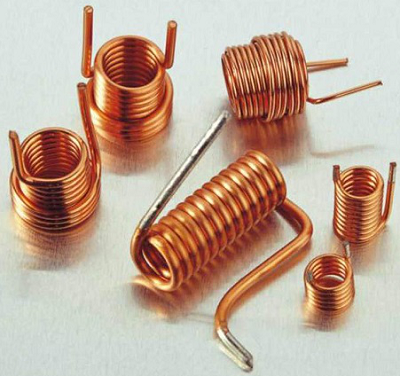An inductor blocks any AC component present in a DC signal. The inductor is sometimes wrapped upon a core, for example a ferrite core. It then looks as in the figure below.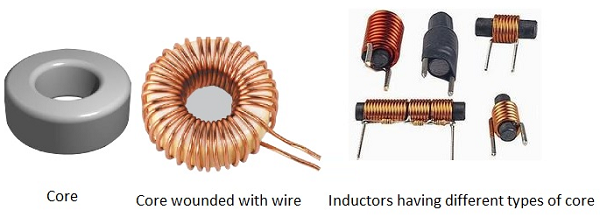The following figure shows an inductor with various parts labelled.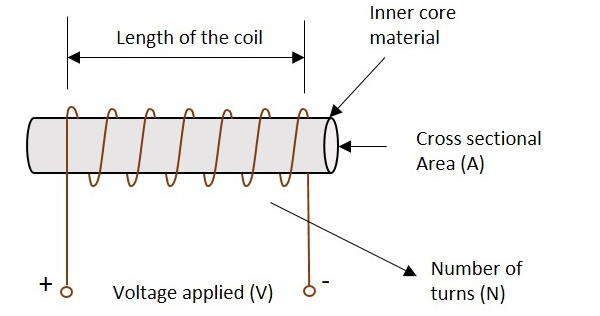### Symbols

The symbols of various types of inductors are as given below.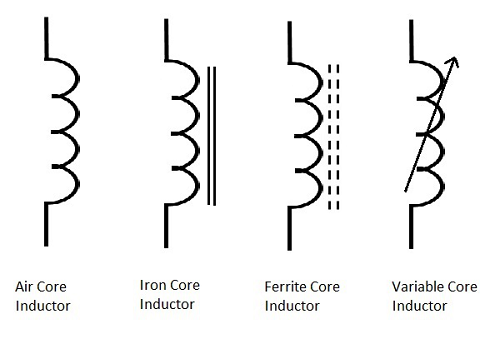### Storage of Energy

One of the Basic properties of electromagnetism is that the current when flows through an inductor, a magnetic field gets created perpendicular to the current flow. This keeps on building up. It gets stabilized at some point, which means that the inductance won’t build up after that. When the current stops flowing, the magnetic field gets decreased.

This magnetic energy gets turned into electrical energy. Hence energy gets stored in this temporarily in the form of magnetic field.

## Working of an Inductor

According to the theory of Electromagnetic Induction, any varying electric current, flowing in a conductor, produces a magnetic field around that, which is perpendicular to the current. Also, any varying magnetic field, produces current in the conductor present in that field, whereas the current is perpendicular to the magnetic field.

Now, if we consider an inductor which is made up of a conducting coil and when some current passes through the inductor, a magnetic field is created perpendicular to it. The following figure indicates an inductor with magnetic field around it.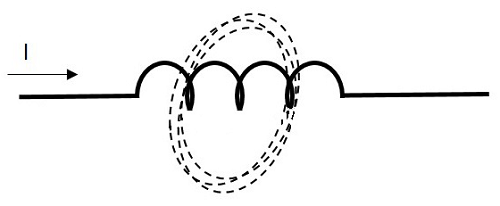Now, here we have a varying magnetic field, which creates some current through the conductor. But this current is produced such that it opposes the main current, which has produced the magnetic field.

If this current is named as Im which means the current produced due to the magnetic field and the magnetic field is indicated by β, the following figure indicates it.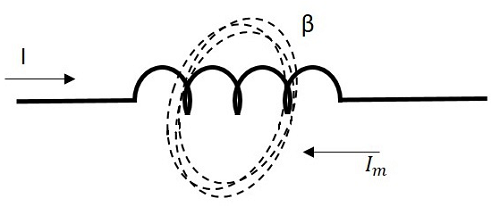This opposing current gains strength with the varying magnetic field, which gains energy by the input supply frequency. Hence as the input current becomes more and more AC with high frequency, the resulting opposing current also gains its strength in opposite direction to the very cause producing it. Now, this opposing current, tries to stop the high frequency AC to pass through the inductor, which means “blocking of AC”.